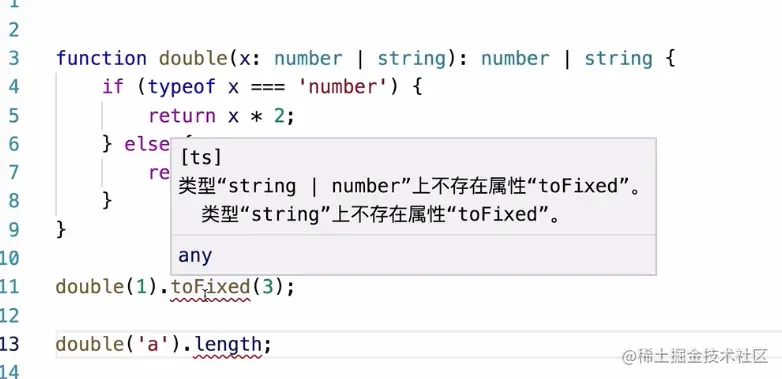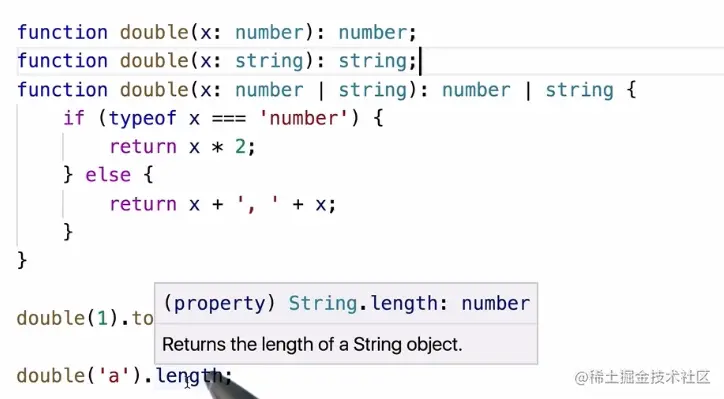# TypeScript 函数的重载

## 函数的重载

``````function double(x: number | string): number | string {
if (typeof x === 'number') {
return x * 2;
} else {
return x + ', ' + x;
}
}````````````function double(x: number): number; // 输入是 number 类型，输出也是 number 类型
function double(x: string): string;
function double(x: number | string): number | string {
if (typeof x === 'number') {
return x * 2;
} else {
return x + ', ' + x;
}
}
let d = double(1);``````### 习题-根据函数重载知识，完善下面代码块

``````function paramType (param: ______): string;
function paramType (param: string): string;
function paramType (param: string | number): string {
return typeof param;
};
paramType('panda');
paramType(10);``````

``````// 函数声明
function paramType (param: ______): string;
function paramType (param: string): string;
// 函数实现
function paramType (param: string | number): string {
return typeof param;
};``````

## 资料-高阶函数

• 接受一个或多个函数作为输入
• 输出一个函数

### 接受一个或多个函数作为输入

``````let \$submitButton = document.querySelector('#submit-button');
});``````

``````let \$submitButton = document.querySelector('#submit-button');
}

### 将函数作为结果返回

``````let replaceSiteUrl = function(text) {
return text.replace(/myblog\.com/ig, 'my-blog.com');
}``````

``````let replaceSiteName = function(text) {
return text.replace(/MySite/ig, 'MyBlog');
}``````

``````let replaceText = function(reg, newText, source){
return function(source) {
return source.replace(reg, newText);
}
}
let replaceSiteUrl = replaceText(/myblog\.com/ig, 'my-blog.com');
console.log(replaceSiteUrl('My site url is https://myblog.com')); // My site url is https://my-blog.com``````